# 12 Logic Programming

Many problems, especially frequent in the field of Artificial Intelligence, and also found elsewhere, e.g., in operations research, are currently solvable only by resorting to some form of search and constraint propagation. Such problems can be specified very concisely, if the programming language abstracts away the details of search by providing don't know nondeterminism. Logic programming and Prolog is considered a suitable formalism for this class of problems. In this chapter we will talk about how to express logic programming and concurrent constraint programming in Oz. In logic programming each procedure can be interpreted as a relation expressed by a logical statement. We will also discuss the relation between Oz and Prolog, and how most Prolog programs have a straight forward translation to Oz programs. For more advanced constraint solving techniques, the reader may look to the companion tutorial on constraint programming in Oz.

Warning:Please note that the material in this chapter is still incomplete.

## 12.1 Constraint Stores

Oz threads share a store where variable bindings are stored in the form of equalities: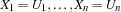where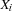are variables and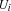are either Oz entities or variables. The constraint store contains the Oz values corresponding to records, numbers, names or variables, and the names that uniquely idententifies the procedures, cells and the various types of chunks (classes, objects, functors, etc.). Conceptually the store is modeled as a conjunctive logical formula: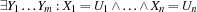, where theare the variables andare Oz values or variables, and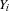are the union of all variables occuring inand. The store is said to be a constraint store. An Oz computation store consists of a constraint store, a procedure store where procedures reside, and a cell store where cells and object states reside.

## 12.2 Computation Spaces

A computation space consists in general of a computation store and a set of executing threads. What we have seen so far is a single computation space. When dealing with logic programming a more elaborate structure will arise with multiple nested computation spaces. The general rules for the structure of computation spaces are as follows.

• There is always a topmost computation space where threads may interact with the external world. A thread trying to add inconsistent constraints (bindings) to the store of the top space will raise a failure exception in the thread. The addition of the inconsistent constraints will be aborted and the constraint store remains always consistent.

• A thread may create a local computation space either directly or indirectly as will be shown in this section. The new computation space will be a child space and the current one the parent space. In general a hierarchy of computation spaces may be created.

• A thread belongs always to one computation space. Also, variables belong to only one computation space.

• A thread in a child space sees and may access variables belonging to its space as well as to all ancestor spaces. The converse is false. A thread in a parent space cannot see the variables of a child space, unless the child space is merged with the parent. In such a case, the child space disappears, and all its content is added to the parent space. The space merge operation may occur due to an explicit operation, or indirectly due to a language construct as will be seen in this section.

• A Thread in a child space my add constraints (bindings) on variables visible to it. This means that it may bind variables belonging to its space or to its ancestor spaces. The binding will only be visible in the current space and all its children spaces if any.

## 12.3 Constraint Entailment and Disentailment

A condition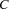is entailed by the storeif, considered as a logical formula, is logically implied by the store, again considered as a logical formula. Intuitively entailment ofmeans that addingto the store does not increase the information already there. Everything is already there.

A conditionis disentailed by the store if the negation ofis logically implied by the store. A disentailed constraint is inconsistent with the information already in the store.

Since a constraint store is a logical formula, we can also talk of a constraint store being entailed, or disentailed by another constraint store. A space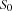is entailed (disentailed) by another space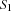if the constraint store ofis entailed (disentailed) by the constraint store if.

We call a space that is disentailed (normally by a parent space) a failed space .

### 12.3.1 Examples

Consider the store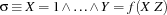and the following conditions:

•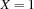is entailed since adding this binding does not increase the information in the store.

•is also entailed. Adding this information does not increase our information. There is a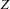that satisfies the above condition. Notice that we do not know which valuewill assume. But whatever value assumed by, the condition would be still satisfied.

•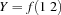is not entailed by the store, since adding this equality increases the information there, namely by making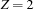.

•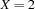or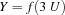are both disentailed since they contradict information already present. They will cause a failure exception to be raised: in the top space this is normally reported to the user in an error message, whereas a subordinated space is merely failed.

## 12.4 Disjunctions

Now we are in a position to understand the nondeterminate constructs of Oz. Oz provides several disjunctive constructs for nondeterminate choice, also known as don't know choice statements.

### 12.4.1 or statement

In all the disjunctive statements we are going to use the notion of a clause and a guard. A clause consists of a guard G and a body S1, and has the following form:

G then S1

The guard G has the form:

 X1 ... Xn in S0

where the variables Xi are existentially quantified with scope extending over both the guard and the body.

The first disjunctive statement has the following form:

or    G1 then S1 [] G2 then S2    ... [] GN then SN end

An or-statement has the following semantics. Assume a thread is executing the statement in space SP.

•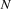spaces are created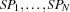withnew threads executing the guards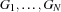.

• Execution of the father thread remains blocked until at most one of the child spaces is not failed.

• If all children spaces are failed, the parent thread raises a failure condition in its space. This means that if the space of the parent thread is the top space, a failure exception is raised. Otherwise the space is local and it becomes a failed space.

• Only one space remains that is not failed which corresponds to the clause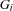then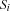. Assume also thathas been reduced to the goal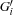and the constraint. In this case, the space is merged with the parent space.and the variables of the store are added to that of the parent store.executes in its own thread, and the original suspending thread resumes executing the statement. This rule of execution is called unit commit in Oz because execution commits to one alternative disjunct (the only one that is left).

### 12.4.2 Shorthand Notation

or ... [] Gi ... end

Stands for

or ... [] Gi then skip ... end

Observe that the or statement does not introduce any don't know nondeterminism. A thread executing such a statement waits until things works out in a determinate course of action.

### 12.4.3 Prolog Comparison

The or statement just described does not have a corresponding construct in Prolog. The Prolog disjunct P ; Q always creates a choice point that is subject to backtracking.

## 12.5 Determinacy Driven Execution

The or-statement of Oz allows a pure logical form of programming style where computations are synchronized by determinacy conditions. Consider the following program.

proc {Ints N Xs}   or N = 0 Xs = nil   [] Xr in        N > 0 = true Xs = N|Xr      {Ints N-1 Xr}   end  end local     proc {Sum3 Xs N R}      or Xs = nil R = N      [] X|Xr = Xs in          {Sum3 Xr X+N R}      end    end in proc {Sum Xs R} {Sum3 Xs 0 R} end end local N S R in    thread {Ints N S} end    thread {Sum S {Browse}} end    N = 1000end

The thread executing Ints will suspend until N is known, because it cannot decide on which disjunct to take. Similarly, Sum3 will wait until the list S is known. S will be defined incrementally and that will lead to the suspension and resumption of Sum3. Things will start to take off when the main thread binds N to 1000. This shows clearly that determinacy driven execution gives the synchronization information need to mimic producer/consumer behavior.

## 12.6 Conditionals

### 12.6.1 Logical Conditional

A logical conditional is a statement having the following form.

cond X1 ... XN in S0 then S1 else S2 end

where Xi are newly introduced variables, and Si are statements. X1 ... XN in S0 then S1 is the clause of the conditional, and S2 is the alternative.

A cond-statement has the following semantics. Assume a thread is executing the statement in space SP.

• A spaceis created, with a single thread executing the guard cond X1 ... XN in S0.

• Execution of the father thread remains blocked untilis either entailed or disentailed. Notice that these conditions may never occur, e. g. when some thread is suspending or running forever in.

• Ifis disentailed, the father thread continues with S2.

• Ifis entailed, assume it has been reduced to the storeand the set of local variables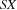In this case, the space is merged with the parent space.andadded to the parent store, and the father thread continues with the execution of S1.

### 12.6.2 Prolog Comparison

The cond statement just described corresponds roughly to Prolog's conditional  P -> Q ; R . Oz is a bit more careful about the scope of variables, so local variables Xi have to be introduced explicitly. cond X in P then Q else R end always has the logical semantics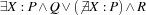, given that we stick to the logical part of Oz. This is not always true in Prolog.

### 12.6.3 Parallel Conditional

A parallel conditional is of the form

cond G1 then S1 [] G2 then S2 ... else SN end

A parallel conditional is executed by evaluating all conditions G1 ... G(N-1) in an arbitrary order, possibly concurrently, each in its own space. If one of the spaces, say Gi, is entailed, its corresponding statement Si is chosen by the father thread. If all spaces are failed, the else statement SN is chosen, otherwise the executing thread suspends.

Parallel conditionals are useful mostly in concurrent programming, e.g. for programming time-out on certain events. This construct is the basic construct in concurrent logic programming languages (also known as committed-choice languages).

As a typical example from concurrent logic programming let us define the indeterministic binary merge, where the arrival timing of elements on the two streams Xs and Ys determines the order of elements on the resulting stream Zs.

proc {Merge Xs Ys Zs}   cond       Xs = nil then Zs = Ys   [] Ys = nil then Zs = Xr   [] X Xr in Xs = X|Xr then Zr in       Zs = X|Zr {Merge Xr Ys Zr}   [] Y Yr in Ys = Y|Yr then Zr in       Zs = Y|Zr {Merge Xs Yr Zr}   end end

In general binary-stream merge is inefficient, specially when multiple of these are used to connect multiple threads to a simple server thread. An efficient way to implement a constant-time multi-merge operator is defined below by using cells and streams instread. The procedure {MMerge STs L} has two arguments STs may be either nil, a list of streams to merged, or of the form merge(ST1 ST2) where each STi is again of the same form as STs.

### 12.7.6 Dynamic Predicates

proc {DisMember X Ys}   dis Ys = X|_ [] Yr in Ys = _|Yr {DisMember X Yr} end end

class DataBase from BaseObject    attr d   meth init       d := {NewDictionary}   end    meth dic($) @d end meth tell(I) case {IsFree I.1} then raise database(nonground(I)) end else Is = {Dictionary.condGet @d I.1 nil} in {Dictionary.put @d I.1 {Append Is [I]}} end end meth ask(I) case {IsFree I} orelse {IsFree I.1} then {DisMember I {Flatten {Dictionary.items @d}}} else {DisMember I {Dictionary.condGet @d I.1 nil}} end end meth entries($)      {Dictionary.entries @d}   end end  declare proc {Dynamic ?Pred}   Pred = {New DataBase init}end proc {Assert P I}   {P tell(I)}end proc {Query P I}   {P ask(I)}end

EdgeP = {Dynamic}{ForAll[edge(1 2) edge(2 1)   % Cycle edge(2 3) edge(3 4) edge(2 5) edge(5 6)       edge(4 6) edge(6 7) edge(6 8) edge(1 5) edge(5 1)  % Cycle]proc {\$ I} {Assert EdgeP I} end }

### 12.7.8 Example: A Simple Expert System

Seif Haridi and Nils Franzén
Version 1.4.0 (20080702)